Question

# In the following reaction, how many grams of H20 are produced if 4.29 g of NzH4...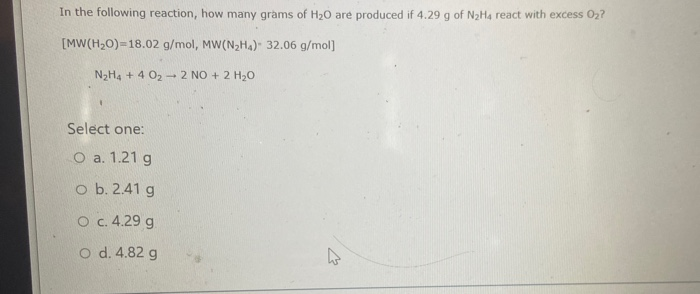In the following reaction, how many grams of H20 are produced if 4.29 g of NzH4 react with excess 02? [MW(H20)=18.02 g/mol, MW(N2Ha). 32.06 g/mol] N H4 + 4 02 - 2 NO + 2 H2O Select one: O a. 1.21 g ob. 2.41g O c.4.29 g o d. 4.82 g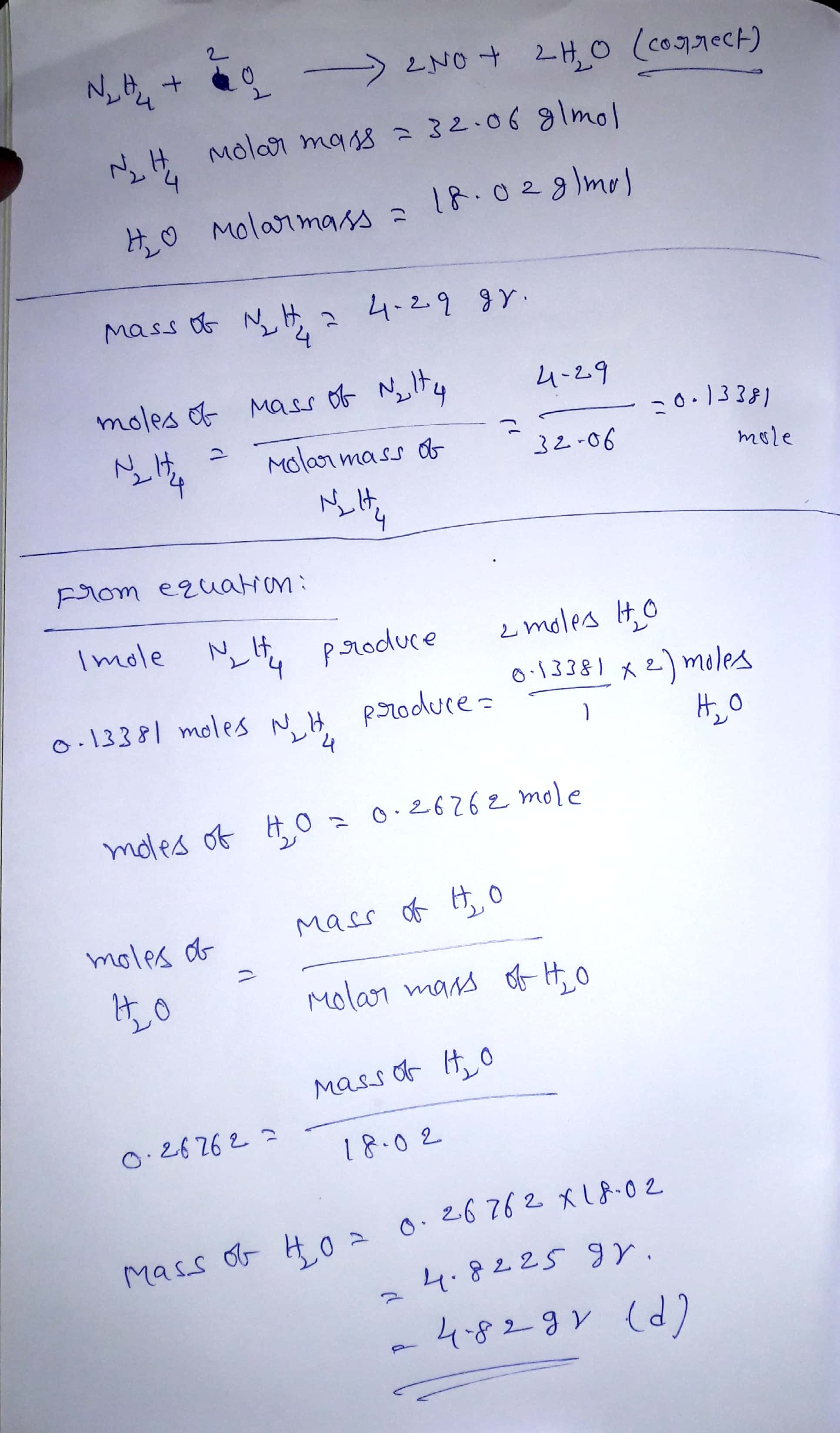#### Earn Coins

Coins can be redeemed for fabulous gifts.

Similar Homework Help Questions
• ### 9. How many grams of CO2 will be produced if 32.10 g of C2H4 is combusted...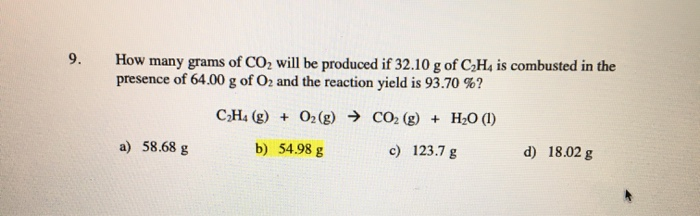9. How many grams of CO2 will be produced if 32.10 g of C2H4 is combusted in the presence of 64.00 g of O2 and the reaction yield is 93.70 % C H4 (g) O2(g) CO2 (g) H2O () a) 58.68 g b) 54.98 g c) 123.7 g d) 18.02 g

• ### Consider the following balanced reaction. How many grams of water are required to form 75.9 g...

Consider the following balanced reaction. How many grams of water are required to form 75.9 g of HNO3? Assume that there is excess NO2 present. The molar masses are as follows: H2O = 18.02 g/mol, HNO3 = 63.02 g/mol. 3 NO2(g) + H2O(l) → 2 HNO3(aq) + NO(g) Answer 26.5 g H2O 10.9 g H2O 43.4 g H2O 21.7 g H2O 38.0 g H2O

• ### d) how many grams of hydrochloric acid are produced when 7.11x1024 molecules of water react? 9....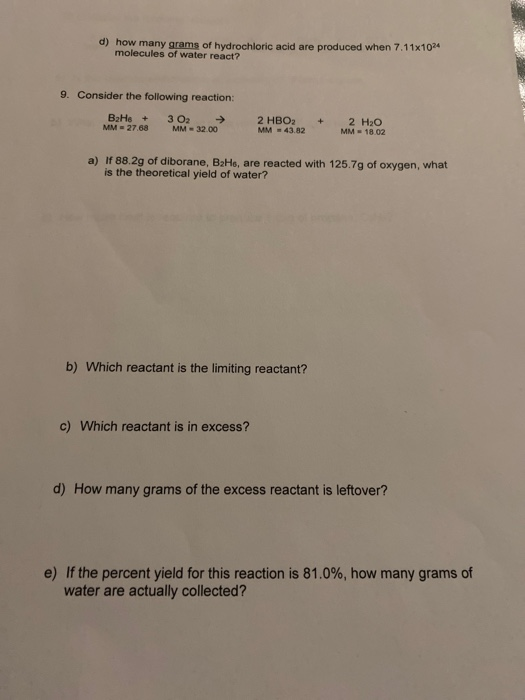d) how many grams of hydrochloric acid are produced when 7.11x1024 molecules of water react? 9. Consider the following reaction: BaHo + MM - 27.68 3 O2 → MM - 32.00 2 HBO2 MM - 43.82 + 2 H2O MM - 18.02 a) If 88.2g of diborane, BaHo, are reacted with 125.7g of oxygen, what is the theoretical yield of water? b) Which reactant is the limiting reactant? c) Which reactant is in excess? d) How many grams of the...

• ### 1. (12 pts) For the balanced equation below, determine how many grams of UF4 (MW: 314.0...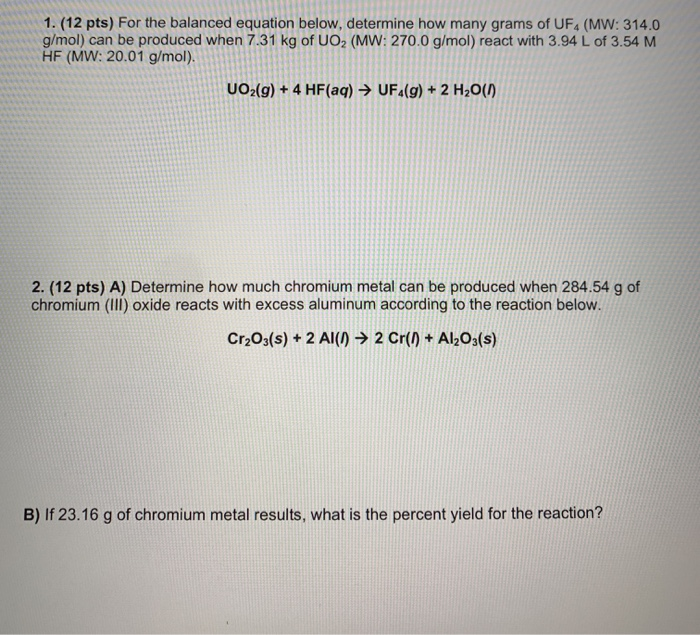1. (12 pts) For the balanced equation below, determine how many grams of UF4 (MW: 314.0 g/mol) can be produced when 7.31 kg of UO2 (MW: 270.0 g/mol) react with 3.94 L of 3.54 M HF (MW: 20.01 g/mol). UO2(g) + 4 HF(aq) → UF4(g) + 2 H20(1) 2. (12 pts) A) Determine how much chromium metal can be produced when 284.54 g of chromium (III) oxide reacts with excess aluminum according to the reaction below. Cr2O3(s) + 2 Al(n)...

• ### 16. How many grams of steam and iron must react to produce 375 g of magnetic...

16. How many grams of steam and iron must react to produce 375 g of magnetic iron oxide, Fe3O4? 3 Fe(s) + 4 H2O(g)  Fe3O4(s) + 4 H2(g) 18. Mercury can be isolated from its ore by the reaction 4 HgS + 4 CaO → 4 Hg + 3 CaS + CaSO4 (a) How many moles of CaS are produced from 2.5 mol CaO? (b) How many grams of Hg are produced along with 9.75 mol of CaSO4? (c) How many...

• ### Consider the following balanced reaction. How many grams of water are required to form 75.9 g...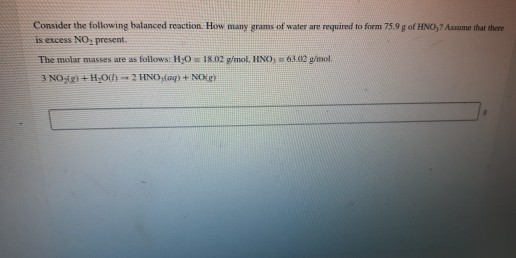Consider the following balanced reaction. How many grams of water are required to form 75.9 g of HNO, Asume that there is excess NO, present. The molar masses are as follows: H 0 18.02 g/mol. HINO, 63.02 g/mol 3 NO2) +H-001) - 2 HNO (aq) + NOC)

• ### How many grams of NO will be produced from 60.7 g of No, reacted with excess...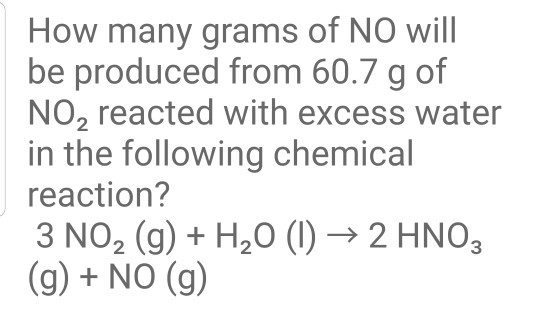How many grams of NO will be produced from 60.7 g of No, reacted with excess water in the following chemical reaction? 3 NO2 (g) + H20 (1) 2 HNO3 (g) + NO (g)

• ### 4) Limiting reagent problem. How many grams of H20 is produced from 40.0 g N204 and...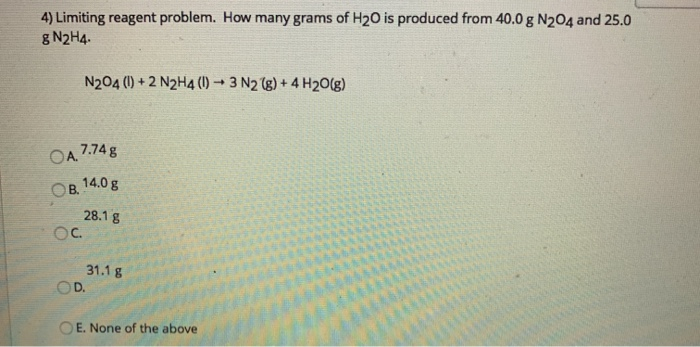4) Limiting reagent problem. How many grams of H20 is produced from 40.0 g N204 and 25.0 g N2H4. N204 (1) + 2 N2H4(0) 3 N2(g) + 4H2O(g) OA 7.74 g OB. 14.0 g 28.1 g OC. 31.18 D. O E. None of the above

• ### Consider the following unbalanced chemical equation. C5H12(/) + 02(g) → CO2(g) + H2O(/) If 37.2 grams...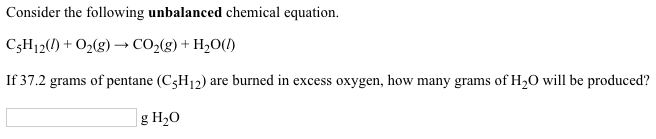Consider the following unbalanced chemical equation. C5H12(/) + 02(g) → CO2(g) + H2O(/) If 37.2 grams of pentane (CH12) are burned in excess oxygen, how many grams of H20 will be produced? g H20

• ### A. Identify the limiting reagent. B. State how many grams of the excess reagent remain. C. State how many grams of product are produced.?

Aspirin (C9H8O4, molecular mass 180.2 g/mol) is synthesized by the reaction of salicylic acid (C7H6O3, molecular mass 138.1 g/mol) with acetic anhydride (C4H6O3, molecular mass 102.1 g/mol) according to the reaction 2 C7H6O3 (s) + C4H6O3(l) → 2C9H8O4(s)+ H2O(l) 2.0g of salicylic acid and 5.4 g of acetic anhydride are mixed and the reaction is allowed to go to completion. Do each of the following: A. Identify the limiting reagent. B. State how many grams of the excess reagent remain....